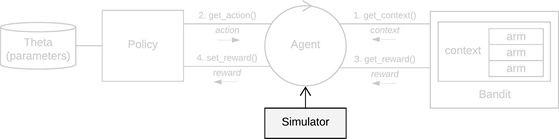The entry point of any contextual simulation.

## Details

A Simulator takes, at a minimum, one or more Agent instances, a horizon (the length of an individual simulation, t = {1, ..., T}) and the number of simulations (How many times to repeat each simulation over t = {1, ..., T}, with a new seed on each repeat*).

It then runs all simulations (in parallel by default), keeping a log of all Policy and Bandit interactions in a History instance.

* Note: to be able to fairly evaluate and compare each agent's performance, and to make sure that simulations are replicable, for each separate agent, seeds are set equally and deterministically for each agent over all horizon x simulations time steps.simulator &lt;- Simulator$new(agents, horizon = 100L, simulations = 100L, save_context = FALSE, save_theta = FALSE, do_parallel = TRUE, worker_max = NULL, set_seed = 0, save_interval = 1, progress_file = FALSE, log_interval = 1000, include_packages = NULL, t_over_sims = FALSE, chunk_multiplier = 1, policy_time_loop = FALSE) ## Arguments agents An Agent instance or a list of Agent instances. horizon integer. The number of pulls or time steps to run each agent, where t = {1, ..., T}. simulations integer. How many times to repeat each agent's simulation over t = {1, ..., T}, with a new seed on each repeat (itself deterministically derived from set\_seed). save_interval integer. Write data to historyonly every save_interval time steps. Default is 1. save_context logical. Save the context matrices X to the History log during a simulation? save_theta logical. Save the parameter list theta to the History log during a simulation? do_parallel logical. Run Simulator processes in parallel? worker_max integer. Specifies how many parallel workers are to be used. If unspecified, the amount of workers defaults to max(workers_available)-1. t_over_sims logical. Of use to, among others, offline Bandits. If t_over_sims is set to TRUE, the current Simulator iterates over all rows in a data set for each repeated simulation. If FALSE, it splits the data into simulations parts, and a different subset of the data for each repeat of an agent's simulation. set_seed integer. Sets the seed of R's random number generator for the current Simulator. progress_file logical. If TRUE, Simulator writes workers_progress.log, agents_progress.log and parallel.log files to the current working directory, allowing you to keep track of respectively workers, agents, and potential errors when running a Simulator in parallel mode. log_interval integer. Sets the log write interval. Default every 1000 time steps. include_packages List. List of packages that (one of) the policies depend on. If a Policy requires an R package to be loaded, this option can be used to load that package on each of the workers. Ignored if do_parallel is FALSE. chunk_multiplier integer By default, simulations are equally divided over available workers, and every worker saves its simulation results to a local history file which is then aggregated. Depending on workload, network bandwith, memory size and other variables it can sometimes be useful to break these workloads into smaller chunks. This can be done by setting the chunk_multiplier to some integer value, where the number of chunks will total chunk_multiplier x number_of_workers. policy_time_loop logical In the case of replay style bandits, a Simulator's horizon equals the number of accepted plus the number of rejected data points or samples. If policy_time_loop is TRUE, the horizon equals the number of accepted data points or samples. That is, when policy_time_loop is TRUE, a Simulator will keep running until the number of data points saved to History is equal to the Simulator's horizon. ## Methods reset() Resets a Simulator instance to its original initialisation values. run() Runs a Simulator instance. history Active binding, read access to Simulator's History instance. ## See also Core contextual classes: Bandit, Policy, Simulator, Agent, History, Plot Bandit subclass examples: BasicBernoulliBandit, ContextualLogitBandit, OfflineReplayEvaluatorBandit Policy subclass examples: EpsilonGreedyPolicy, ContextualLinTSPolicy ## Examples if (FALSE) { policy <- EpsilonGreedyPolicy$new(epsilon = 0.1)
bandit    <- BasicBernoulliBandit$new(weights = c(0.6, 0.1, 0.1)) agent <- Agent$new(policy, bandit, name = "E.G.", sparse = 0.5)

history   <- Simulator$new(agents = agent, horizon = 10, simulations = 10)$run()

summary(history)

plot(history)

dt <- history$get_data_table() df <- history$get_data_frame()

print(history$cumulative$E.G.$cum_regret_sd) print(history$cumulative$E.G.$cum_regret)

}